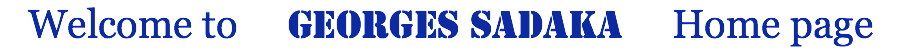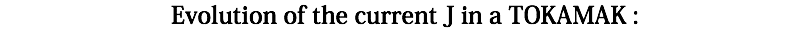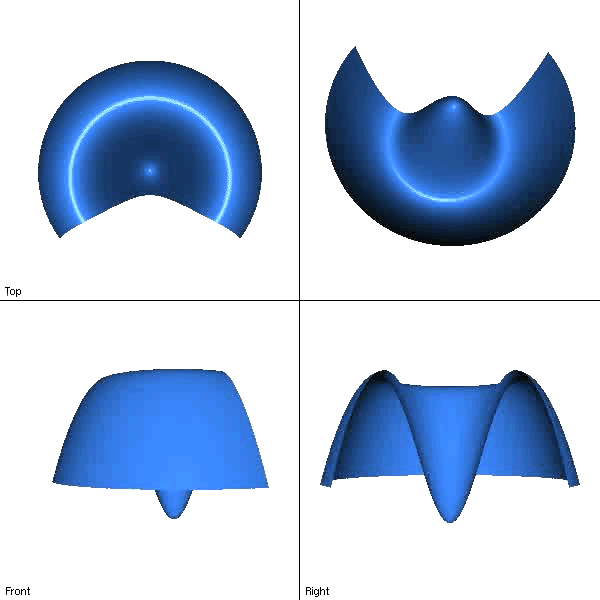During CEMRACS'10, dedicated to numerical models for the fusion, I participated in the project EMAFF (Magnetic Equations with FreeFem++), see Després & al. The goal of this project is to evaluate the FreeFem++ tool on basic magnetic equations arising in Fusion Plasma in the context of the ITER project, especially to capture the isoline of the magnetic potential with enough accuracy because they represent the free surface of the plasma inside the tokamak. We first considered the Grad Shafranov equation which describes the equilibrium geometry in axisymmetric. We tested the solver for different analytical solutions, first for solutions of the equation of equilibrium with Soloviev, then in the general case of a nonlinear polynomial RHS. Then we studied numerical schemes for the problem of Current Hole in cylindrical geometry :where the Poisson bracket, denoted by [.,.], is given by :We used in this simulation P1 finite element in space and Crank-Nicolson scheme in time.